# DICE REASONING: Concepts & tricks

Basic Concepts:
What is a Dice?
A Dice is a cube. In a cube, there are six faces. Let us study some basic facts about Cube using the given figure.
The six faces in the cube are– ABCG, GCDE, DEFH, BCDH, AGEF and ABHF.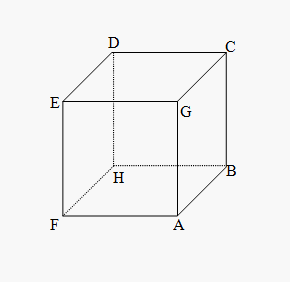1. Four faces are adjacent to one face
2. There are pairs of opposite faces e.g. Opposite of DEFH is ABCG and so on
3. CDEG is the upper face of the cube
4. ABHF is the bottom face of the cube
Important Facts:
1.  A cube has 6 square faces or sides
2. A cube has 8 points (vertices)
3. A cube has 12 edges
4. Only 3 sides of a cube are visible at a time (known as “Joint Sides”) and these sides can never be on the opposite side of each other
5. Things that are shaped like a cube are often referred to as ‘cubic’
6. Most dice are cube shaped, with the numbers 1 to 6 on the different faces.
Certain Basic Rules:
There are certain dice rules in reasoning which can be sued to solve dice-based questions:
Rule No. 1:
Two opposite faces of the dice cannot be adjacent to each other.
E.g. Two positions of a dice are shown below.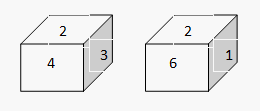Here, faces with number 4, 3, 6 and 1 are adjacent to the face number 2.
Therefore, the 4,3,6,1 can’t be opposite to the face number 2.
Therefore, face number 5  is opposite to the face number 2.
Rule No. 2:
If two dice are shown as below, and one of the two common faces (Face number 4) is in the same position, then the remaining faces will be opposite to each other.
E.g.: Two dice are shown below.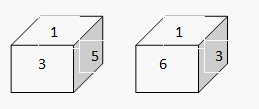In both the diagrams, two faces numbered 1 & 3 are common.
Also, 5 & 6 are remaining faces. Hence, face which is number 5 is opposite to the face number 6.
Rule No. 3:
If in 2 different positions of the dice, the positions (different), the position of the face that’s common is the same, and then the opposite faces of the faces that remain will be in the same positions.
E.g.: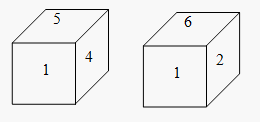In both the positions, face number 1 is common for both dice is same.
Therefore, the opposite of 4 is 2 and the opposite of 5 is 6.
Rule No. 4:
If 2 positions of a die are given (Different) and it is also stated that common face is different then the face opposite to the given common face would be that which is not shown on any given face in the 2 given positions. It is also to be noted that the opposite face of the faces that are left cannot be the same.
E.g.: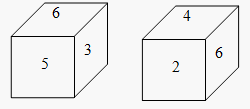Note, in the above shown dice, the face having value 6 is not in the similar position.
The face numbered 1 is not shown.
So, the face opposite to the face with number 6 is 1.
Also, the opposite face of 3 is the face with number 2 and the opposite to face numbered 5 is the face with number 1.
Dice Reasoning Tricks: Deconstructed Cube
Deconstructing a cube:
When we are given a dice it is somewhat difficult to visualize it in 3-D so what we do is that we flatten the cube. We can form a cube that has been flattened where we can visualize, the square at the farthest end will give the top of the cube and the square that is the middle will form the base of the given dice. The given figures below can help you understand the theory stated above.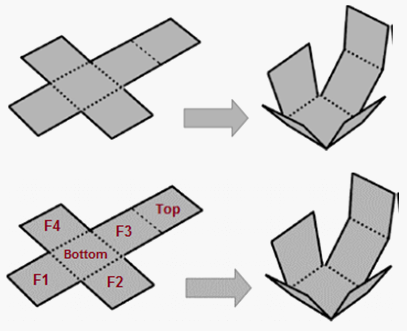The rest of the square will give the adjacent sides of the dice. Note that we have to clearly visualize the adjacent sides and we have to figure out what exactly the question is asking. The flattening of dice is the easiest way that we can use to solve the dice problems.
Now that you know the basic concepts on cubes and dice reasoning tricks. Let us study a few solved examples on dice reasoning Click here & solve some questions on dice.